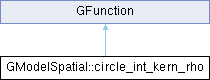GammaLib  1.7.0.dev
GModelSpatial::circle_int_kern_rho Class Reference

#include <GModelSpatial.hpp>

Inheritance diagram for GModelSpatial::circle_int_kern_rho:## Public Member Functions

circle_int_kern_rho (const GModelSpatial *model, const GSkyRegionCircle *reg, const GEnergy &srcEng, const GTime &srcTime, const double distance, const double cosdist, const double sindist, const double modrad, const double cosmodrad)

double eval (const double &rho)
Kernel for circular sky region radial integration. More...Public Member Functions inherited from GFunction
GFunction (void)
Void constructor. More...

GFunction (const GFunction &function)
Copy constructor. More...

virtual ~GFunction (void)
Destructor. More...

GFunctionoperator= (const GFunction &function)
Assignment operator. More...

## Public Attributes

const GModelSpatialm_model
Spatial model. More...

const GSkyRegionCirclem_reg
Interation region. More...

GEnergy m_srcEng
Photon energy. More...

GTime m_srcTime
Photon time. More...

double m_dist

double m_cosdist
Cos of distance model-region. More...

double m_sindist
Sin of distance model-region. More...Protected Member Functions inherited from GFunction
void init_members (void)
Initialise class members. More...

void copy_members (const GFunction &function)
Copy class members. More...

void free_members (void)
Delete class members. More...

## Detailed Description

Definition at line 127 of file GModelSpatial.hpp.

## Constructor & Destructor Documentation

 GModelSpatial::circle_int_kern_rho::circle_int_kern_rho ( const GModelSpatial * model, const GSkyRegionCircle * reg, const GEnergy & srcEng, const GTime & srcTime, const double distance, const double cosdist, const double sindist, const double modrad, const double cosmodrad )
inline

Definition at line 129 of file GModelSpatial.hpp.

## Member Function Documentation

 double GModelSpatial::circle_int_kern_rho::eval ( const double & rho )
virtual

Kernel for circular sky region radial integration.

Parameters
Returns
Integration kernel defined as

$\int_{\rho_{\rm min}}^{\rho_{\rm max}} K(\rho | E, t) d\rho$

of a spatial model over a circular region. The eval() method computes

$K(\rho | E, t) = \sin \rho \times \int_{\omega} M(\rho, \omega | E, t) d\omega$

where $$M(\rho, \omega | E, t)$$ is the spatial model, $$\rho$$ is the distance from the region centre, and $$\omega$$ is the position angle with respect to the connecting line between the region centre and the direction on the sky.

Implements GFunction.

Definition at line 498 of file GModelSpatial.cpp.

## Member Data Documentation

 double GModelSpatial::circle_int_kern_rho::m_cosdist

Cos of distance model-region.

Definition at line 154 of file GModelSpatial.hpp.

Referenced by eval().

Definition at line 157 of file GModelSpatial.hpp.

Referenced by eval().

 double GModelSpatial::circle_int_kern_rho::m_dist

Definition at line 153 of file GModelSpatial.hpp.

Referenced by eval().

 const GModelSpatial* GModelSpatial::circle_int_kern_rho::m_model

Spatial model.

Definition at line 149 of file GModelSpatial.hpp.

Referenced by eval().

Definition at line 156 of file GModelSpatial.hpp.

Referenced by eval().

 const GSkyRegionCircle* GModelSpatial::circle_int_kern_rho::m_reg

Interation region.

Definition at line 150 of file GModelSpatial.hpp.

Referenced by eval().

 double GModelSpatial::circle_int_kern_rho::m_sindist

Sin of distance model-region.

Definition at line 155 of file GModelSpatial.hpp.

Referenced by eval().

 GEnergy GModelSpatial::circle_int_kern_rho::m_srcEng

Photon energy.

Definition at line 151 of file GModelSpatial.hpp.

Referenced by eval().

 GTime GModelSpatial::circle_int_kern_rho::m_srcTime

Photon time.

Definition at line 152 of file GModelSpatial.hpp.

Referenced by eval().

The documentation for this class was generated from the following files: